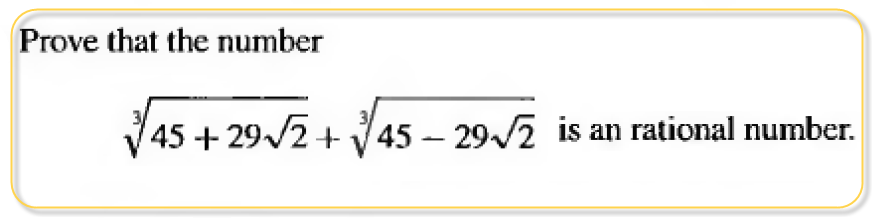User Name Remember Me? Password

 Algebra Pre-Algebra and Basic Algebra Math Forum

 June 22nd, 2014, 01:24 AM #1 Member   Joined: Jun 2014 From: Math Forum Posts: 67 Thanks: 4 rationalJune 22nd, 2014, 05:53 AM #2 Global Moderator   Joined: Dec 2006 Posts: 21,036 Thanks: 2274 $\displaystyle \sqrt[\large3]{45+29\sqrt2} + \sqrt[\large3]{45-29\sqrt2} = (3 + \sqrt2) + (3 - \sqrt2) = 6$June 22nd, 2014, 06:05 AM #3 Senior Member   Joined: Feb 2010 Posts: 714 Thanks: 151 Let $\displaystyle A= \sqrt{x} + \sqrt{y}$ where $\displaystyle x=45+29\sqrt{2}$ and $\displaystyle y=45-29\sqrt{2}$. Note that $\displaystyle x+y=90$, $\displaystyle xy=2025-841 \cdot 2=343=7^3$ Then $\displaystyle A^3 = (\sqrt{x} + \sqrt{y})^3$ $\displaystyle A^3 = x + 3\sqrt{x^2y}+3\sqrt{xy^2}+y$ $\displaystyle A^3 = (x+y) + 3\sqrt{xy}(\sqrt{x} + \sqrt{y})$ $\displaystyle A^3 = 90 + 21A$ $\displaystyle A^3 - 21A - 90=0$ This equation has two complex roots and one real real. Using the rational roots theorem we find that the only real root is 6. Thus $\displaystyle \sqrt{45+29\sqrt{2}} + \sqrt{45-29\sqrt{2}}=6$ and is therefore a rational number.Tags rationalThread ToolsShow Printable VersionEmail this Page Display ModesLinear ModeSwitch to Hybrid ModeSwitch to Threaded ModeSimilar Threads Thread Thread Starter Forum Replies Last Post LucasPR Abstract Algebra 13 May 1st, 2013 11:32 PM drewm Algebra 4 July 2nd, 2011 04:02 PM stuart clark Algebra 1 May 19th, 2011 12:14 AM lepeisi Abstract Algebra 3 March 30th, 2011 01:00 PM advancedfunctions Calculus 2 April 6th, 2010 12:41 PM

 Contact - Home - Forums - Cryptocurrency Forum - Top